# 2 2 7 3 Factoring xbx bxcc Warm

• Slides: 14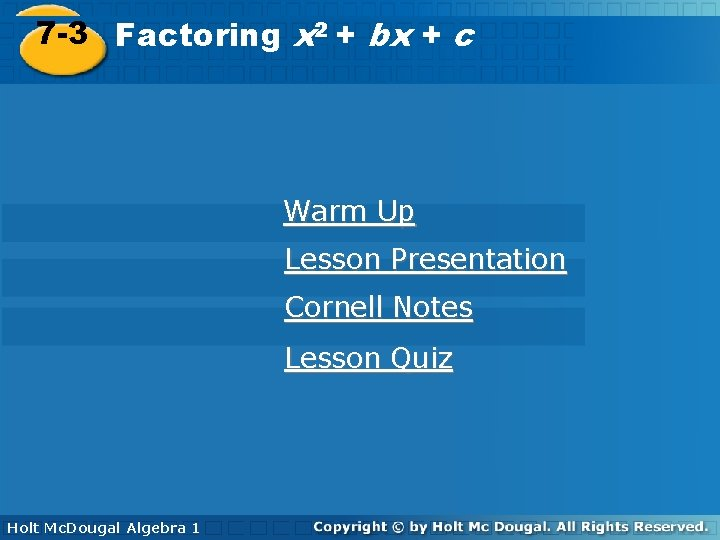2 2 7 -3 Factoring x++bx bx++cc Warm Up Lesson Presentation Cornell Notes Lesson Quiz Holt Algebra 1 Holt Mc. Dougal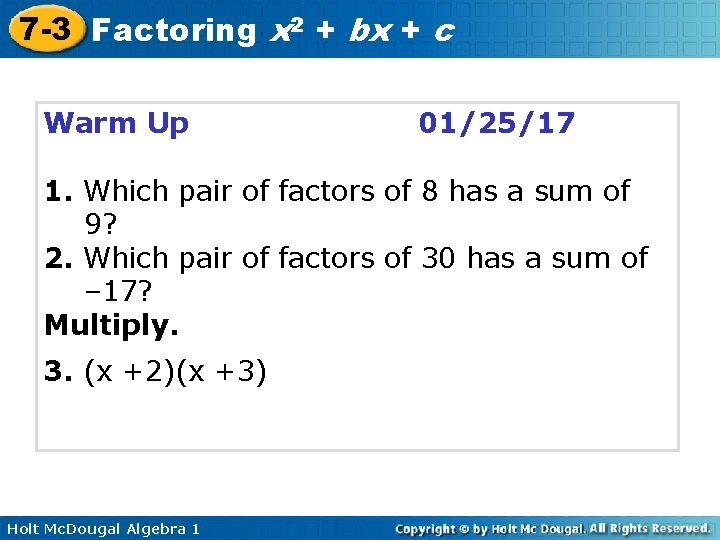7 -3 Factoring x 2 + bx + c Warm Up 01/25/17 1. Which pair of factors of 8 has a sum of 9? 2. Which pair of factors of 30 has a sum of – 17? Multiply. 3. (x +2)(x +3) Holt Mc. Dougal Algebra 1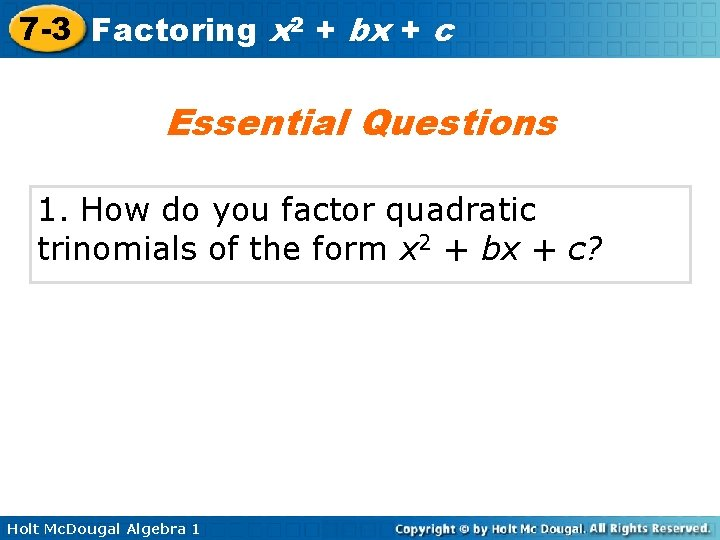7 -3 Factoring x 2 + bx + c Essential Questions 1. How do you factor quadratic trinomials of the form x 2 + bx + c? Holt Mc. Dougal Algebra 1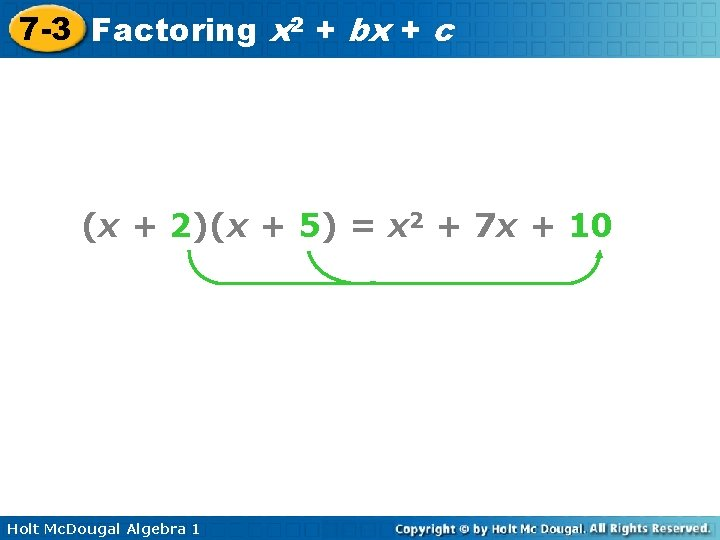7 -3 Factoring x 2 + bx + c (x + 2)(x + 5) = x 2 + 7 x + 10 Holt Mc. Dougal Algebra 1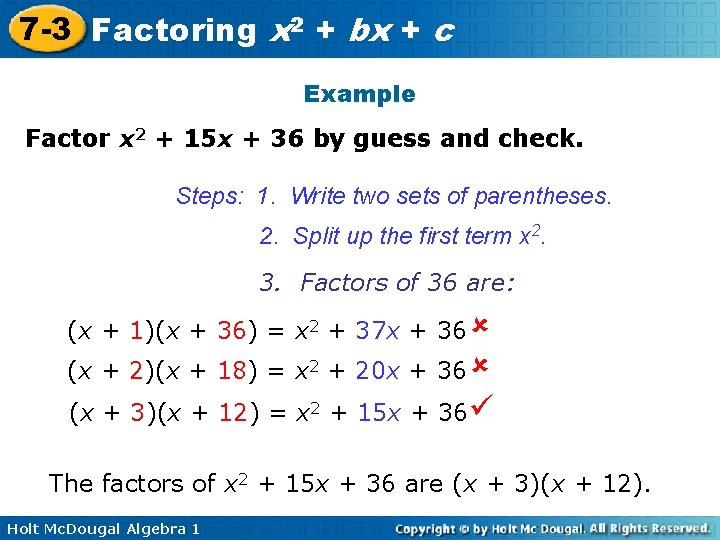7 -3 Factoring x 2 + bx + c Example Factor x 2 + 15 x + 36 by guess and check. Steps: 1. Write two sets of parentheses. 2. Split up the first term x 2. 3. Factors of 36 are: (x + 1)(x + 36) = x 2 + 37 x + 36 (x + 2)(x + 18) = x 2 + 20 x + 36 (x + 3)(x + 12) = x 2 + 15 x + 36 The factors of x 2 + 15 x + 36 are (x + 3)(x + 12). Holt Mc. Dougal Algebra 1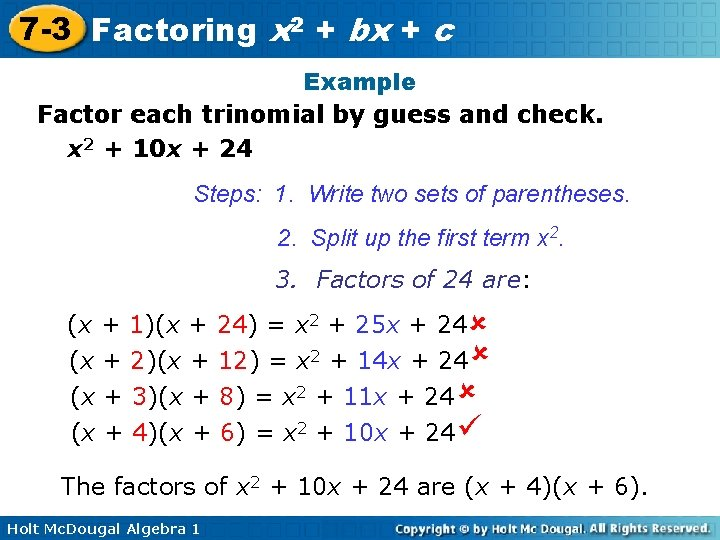7 -3 Factoring x 2 + bx + c Example Factor each trinomial by guess and check. x 2 + 10 x + 24 Steps: 1. Write two sets of parentheses. 2. Split up the first term x 2. 3. Factors of 24 are: (x + 1)(x + 24) = x 2 + 25 x + 24 (x + 2)(x + 12) = x 2 + 14 x + 24 (x + 3)(x + 8) = x 2 + 11 x + 24 (x + 4)(x + 6) = x 2 + 10 x + 24 The factors of x 2 + 10 x + 24 are (x + 4)(x + 6). Holt Mc. Dougal Algebra 1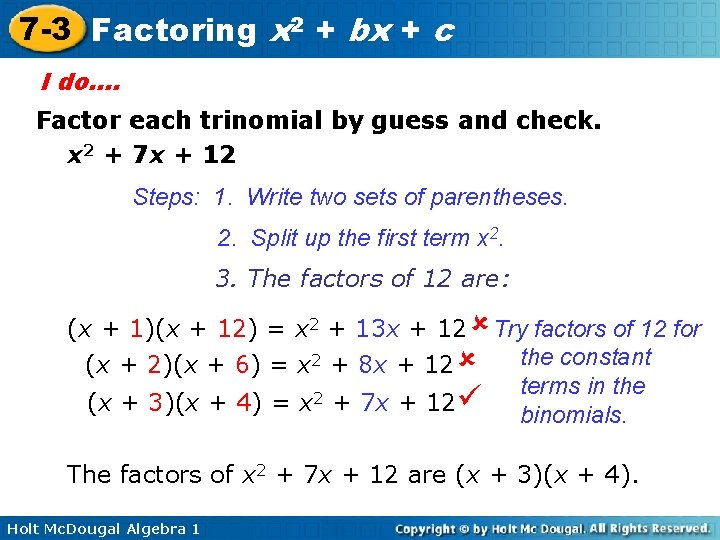7 -3 Factoring x 2 + bx + c I do…. Factor each trinomial by guess and check. x 2 + 7 x + 12 Steps: 1. Write two sets of parentheses. 2. Split up the first term x 2. 3. The factors of 12 are: (x + 1)(x + 12) = x 2 + 13 x + 12 Try factors of 12 for the constant (x + 2)(x + 6) = x 2 + 8 x + 12 terms in the 2 (x + 3)(x + 4) = x + 7 x + 12 binomials. The factors of x 2 + 7 x + 12 are (x + 3)(x + 4). Holt Mc. Dougal Algebra 17 -3 Factoring x 2 + bx + c x 2 12 (x + 3)(x +4) = x 2 + 7 x + 12 3 x 4 x Holt Mc. Dougal Algebra 1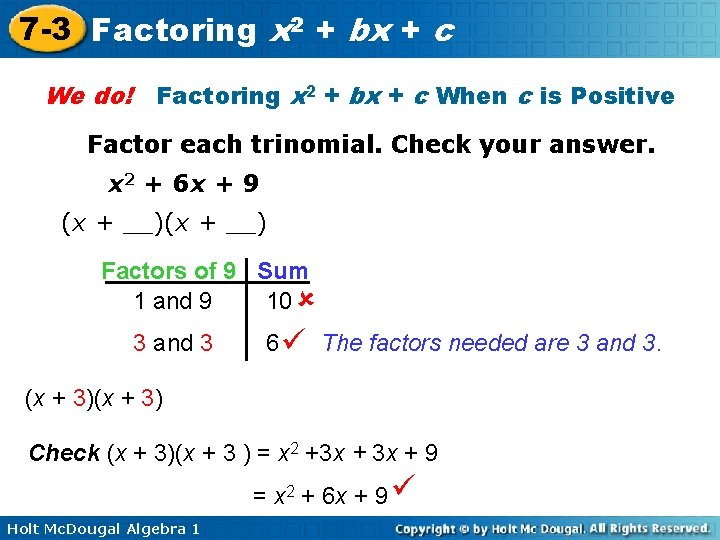7 -3 Factoring x 2 + bx + c We do! Factoring x 2 + bx + c When c is Positive Factor each trinomial. Check your answer. x 2 + 6 x + 9 (x + __) Factors of 9 Sum 1 and 9 10 3 and 3 6 The factors needed are 3 and 3. (x + 3) Check (x + 3)(x + 3 ) = x 2 +3 x + 9 = x 2 + 6 x + 9 Holt Mc. Dougal Algebra 1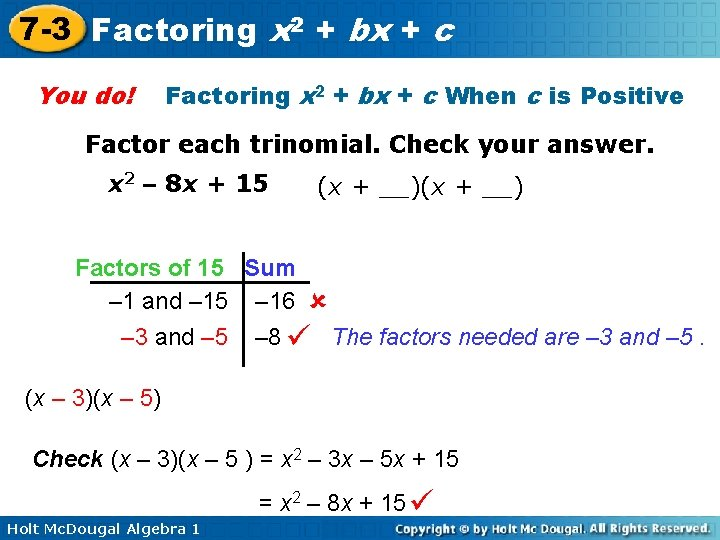7 -3 Factoring x 2 + bx + c You do! Factoring x 2 + bx + c When c is Positive Factor each trinomial. Check your answer. x 2 – 8 x + 15 (x + __) Factors of 15 Sum – 1 and – 15 – 16 – 3 and – 5 – 8 The factors needed are – 3 and – 5. (x – 3)(x – 5) Check (x – 3)(x – 5 ) = x 2 – 3 x – 5 x + 15 Holt Mc. Dougal Algebra 1 = x 2 – 8 x + 15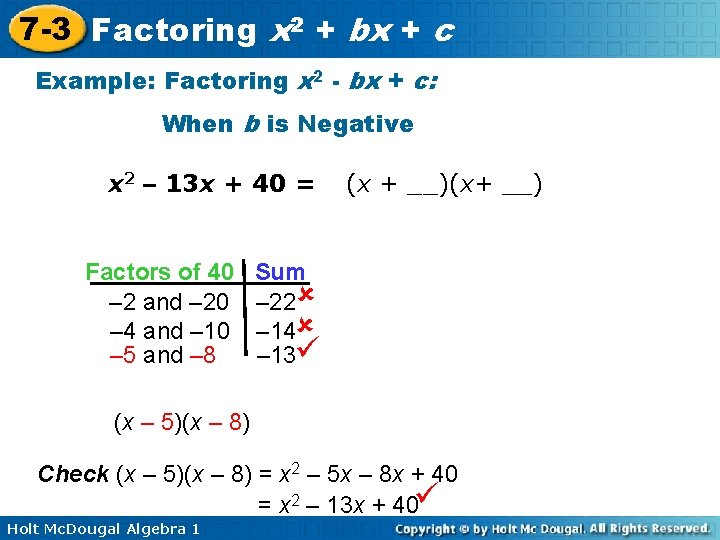7 -3 Factoring x 2 + bx + c Example: Factoring x 2 - bx + c: When b is Negative x 2 – 13 x + 40 = Factors of 40 – 2 and – 20 – 4 and – 10 – 5 and – 8 (x + __)(x+ __) Sum – 22 – 14 – 13 (x – 5)(x – 8) Check (x – 5)(x – 8) = x 2 – 5 x – 8 x + 40 = x 2 – 13 x + 40 Holt Mc. Dougal Algebra 1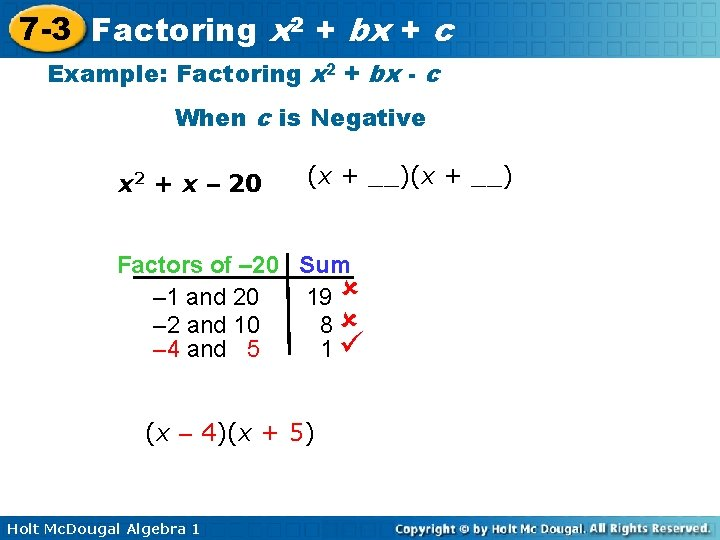7 -3 Factoring x 2 + bx + c Example: Factoring x 2 + bx - c When c is Negative x 2 + x – 20 (x + __) Factors of – 20 Sum – 1 and 20 19 – 2 and 10 8 – 4 and 5 1 (x – 4)(x + 5) Holt Mc. Dougal Algebra 1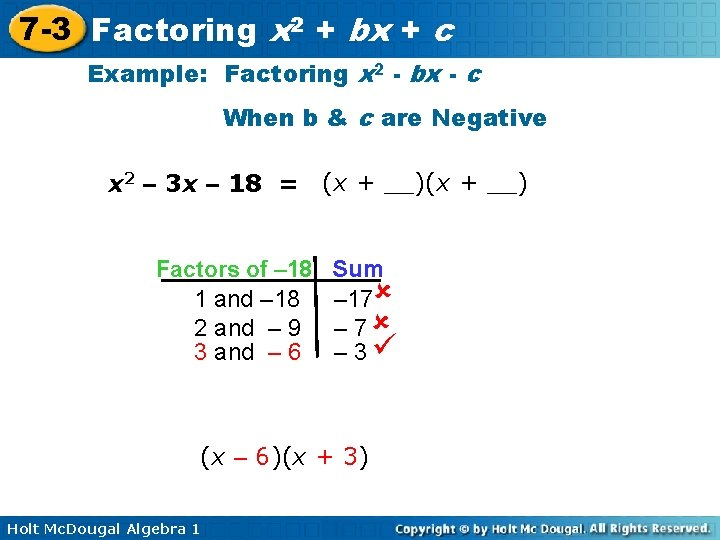7 -3 Factoring x 2 + bx + c Example: Factoring x 2 - bx - c When b & c are Negative x 2 – 3 x – 18 = (x + __) Factors of – 18 1 and – 18 2 and – 9 3 and – 6 Sum – 17 – 3 (x – 6)(x + 3) Holt Mc. Dougal Algebra 1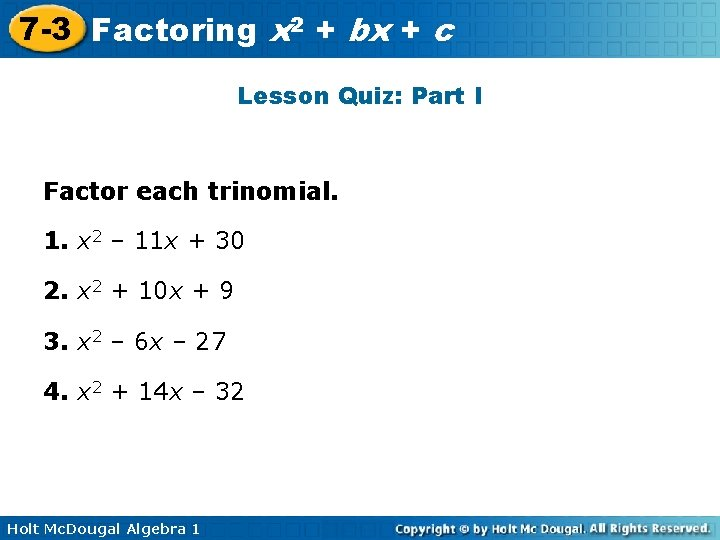7 -3 Factoring x 2 + bx + c Lesson Quiz: Part I Factor each trinomial. 1. x 2 – 11 x + 30 2. x 2 + 10 x + 9 3. x 2 – 6 x – 27 4. x 2 + 14 x – 32 Holt Mc. Dougal Algebra 1